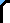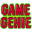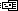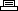Cheats DS GBA PC PS2 PS3 PSP Wii Xbox Xbox 360 New ReviewsSite Search

This Site Web
 Home: Game Cheats: Nintendo: Game GenieBest of the Best Championship KarateEmail this Page  |Printer Friendly Version

```AANIGYPA + VTNIPYSA     Each round is 0:10 instead of 1:00
AANIGYPA + OZVSYYSE + ZANIANTI  Each round is 0:20
AANIGYPA + OZVSYYSE + LANIANTI  Each round is 0:30
AANIGYPA + OZVSYYSE + GANIANTI  Each round is 0:40
AANIGYPA + OZVSYYSE + IANIANTI  Each round is 0:50
ZANIGYPA        Each round is 2:00
LANIGYPA        Each round is 3:00
GANIGYPA        Each round is 4:00
IANIGYPA        Each round is 5:00
TANIGYPA        Each round is 6:00
YANIGYPA        Each round is 7:00
AANIGYPE        Each round is 8:00
PANIGYPE        Each round is 9:00
PAOSUZIA        Each match is 1 round instead of 5
ZAOSUZIA        Each match is 2 rounds
LAOSUZIA        Each match is 3 rounds
GAOSUZIA        Each match is 4 rounds
TAOSUZIA        Each match is 6 rounds
SXVSAZVG        Infinite time (round never ends)